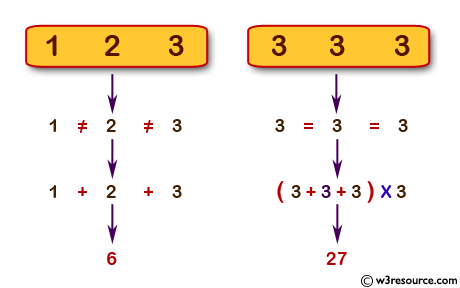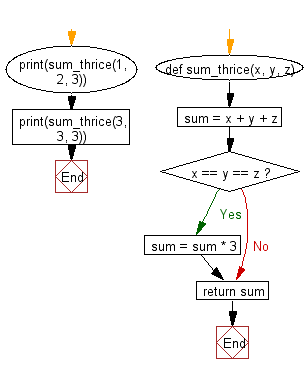﻿ Python: Calculate the sum of three given numbers, if the values are equal then return thrice of their sum - w3resource# Python: Calculate the sum of three given numbers, if the values are equal then return thrice of their sum

## Python Basic: Exercise-18 with Solution

Write a Python program to calculate the sum of three given numbers, if the values are equal then return thrice of their sum.

Pictorial Presentation:Sample Solution:-

Python Code:

``````def sum_thrice(x, y, z):

sum = x + y + z

if x == y == z:
sum = sum * 3
return sum

print(sum_thrice(1, 2, 3))
print(sum_thrice(3, 3, 3))
```
```

Sample Output:

```6
27
```

Flowchart:## Visualize Python code execution:

The following tool visualize what the computer is doing step-by-step as it executes the said program:

Python Code Editor:

Have another way to solve this solution? Contribute your code (and comments) through Disqus.

What is the difficulty level of this exercise?

Test your Python skills with w3resource's quiz

﻿

## Python: Tips of the Day

Try-catch-else construct:

```try:
foo()
except Exception:
print("Exception occured")
else:
print("Exception didnt occur")
finally:
print("Always gets here")
```